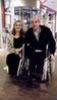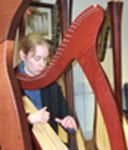## Hugging the world

Your platform. Get to know each other (but don't forget about us!)

Moderator: All Moderators

Happy Easter!!!! ((((((((((((((((Mise, Turlough and the rest of the forum))))))))))))))
Dave

From Wales...via Birmingham!!!!Dab051176

Posts: 1299
Joined: Wed Mar 05, 2008 1:00 pm
Location: Flintshire, North Wales

(((((((((((((((((Easter hug for everybody))))))))))))))))))))))

Happy belated Easter dear fellow forummers! Just got back to the computer zone again from the countryside, but better late than never!

Suvi
"Your golden breath took darkness away, if I ask, You won't let me go..."cantilena91

Posts: 12992
Joined: Fri Sep 05, 2003 6:33 am
Location: city of Oulu, Finland

(((((((((((((((((((Forum))))))))))))))))))))))

Belated happy Easter!Music and friendship - the answer to life's problems.

Whisper to the wild water
Step By Step
Slowly I turn - Máire BrennanGrania
Moderator

Posts: 11209
Joined: Tue Aug 31, 2004 2:26 pm
Location: England

{{{{{{{{{{G}}}}}}}}}} - apologies for not being around like .... forever??!

{{{{{Forum}}}}} - A general hurgIf you don't know what eschatology is then don't worry; it's not the end of the world.
Purveyor of fine sarcasm since 1966.
Angoid

Posts: 3678
Joined: Tue Mar 02, 2004 10:08 pm
Location: In the cave

((((((((((((((((((G)))))))))))))))) Good to see you around, and thanks for the Email - should be superb craic!((((((((((((((Angus))))))))))))))) Great to see you, too - and I'll avoid twee clichés about it having been "too long"!!!!::wink:
Dave

From Wales...via Birmingham!!!!Dab051176

Posts: 1299
Joined: Wed Mar 05, 2008 1:00 pm
Location: Flintshire, North Wales

{{{{{{{{{{Dave}}}}}}}}}} - but it has, and for that I apologise!
If you don't know what eschatology is then don't worry; it's not the end of the world.
Purveyor of fine sarcasm since 1966.
Angoid

Posts: 3678
Joined: Tue Mar 02, 2004 10:08 pm
Location: In the cave

(((((((((((((((((((((((((Angus))))))))))))))))))))))))))))))))) Great to see you! Keep talking to me!

(((((((((((((((((((Dave))))))))))))))))))))))) Superb craic doesn't even begin to cover itMusic and friendship - the answer to life's problems.

Whisper to the wild water
Step By Step
Slowly I turn - Máire BrennanGrania
Moderator

Posts: 11209
Joined: Tue Aug 31, 2004 2:26 pm
Location: England

{{{{{{{{{{G}}}}}}}}}} - Will dowe must touch base properly before too much longerIf you don't know what eschatology is then don't worry; it's not the end of the world.
Purveyor of fine sarcasm since 1966.
Angoid

Posts: 3678
Joined: Tue Mar 02, 2004 10:08 pm
Location: In the cave

(((((((((((((((((((T with the Maggies))))))))))))))) For the great gig last night!((((((((((((((((((Tim)))))))))))))))))) For putting up with me bombarding you with questions about Gweedore in June!!!!!!and, of course......

((((((((((((((((((((((((((((((((((((((Moya)))))))))))))))))))))))))))) As ever, for the chat afterwards!EDIT (to save a double post!): ((((((((((((((((((Angus & Thea)))))))))))))) Happy belated anniversary. Hope you had a great time in Dublin!Dave

From Wales...via Birmingham!!!!Dab051176

Posts: 1299
Joined: Wed Mar 05, 2008 1:00 pm
Location: Flintshire, North Wales

((((((((((((((((((((((Forum folk)))))))))))))))))))))))))))

HelloIt's been a while.
We shall not cease from exploration
And the end of all our exploring
Will be to arrive where we started
And know the place for the first time.
-T. S. Eliot, 'Little Gidding' Vnessieq
Moderator

Posts: 10856
Joined: Sat Oct 11, 2003 5:58 pm
Location: Cambridge/Belfast

nessieq wrote: HelloIt's been a while.

And the prize for Understatement of the Year goes to.....((((((((((((((((((((((((((Ness)))))))))))))))))))))))))))))) Good to see you!
Dave

From Wales...via Birmingham!!!!Dab051176

Posts: 1299
Joined: Wed Mar 05, 2008 1:00 pm
Location: Flintshire, North Wales

((((((((((((((Ness))))))))))))))) Good to see you here!and good luck with taking the right decisions((((((((((((((Dave))))))))))))))) for all the help and so many great chats!Luned

Posts: 1018
Joined: Sat Mar 24, 2007 5:19 pm
Location: Poland

Luned wrote:((((((((((((((Dave))))))))))))))) for all the help and so many great chats!And here's to many more! ((((((((((((((Luned)))))))))))))))

More hugs for:

(((((((((((((((((((((((T with the Maggies))))))))))))))))))))))))) For another awesome night in Letterkenny last night.

(((((((((((((((((((Tim)))))))))))))))))))))))))) For all the help sorting the logistics when I found out the lift at the theatre was kaput!(((((((((((((((((((((((((((((((Moya)))))))))))))))))))))))))))) No explanation required!Dave

From Wales...via Birmingham!!!!Dab051176

Posts: 1299
Joined: Wed Mar 05, 2008 1:00 pm
Location: Flintshire, North Wales

Dab051176 wrote:
Luned wrote:((((((((((((((Dave))))))))))))))) for all the help and so many great chats!And here's to many more! ((((((((((((((Luned)))))))))))))))

More hugs for:

(((((((((((((((((((((((T with the Maggies))))))))))))))))))))))))) For another awesome night in Letterkenny last night.

(((((((((((((((((((Tim)))))))))))))))))))))))))) For all the help sorting the logistics when I found out the lift at the theatre was kaput!(((((((((((((((((((((((((((((((Moya)))))))))))))))))))))))))))) No explanation required!EDIT to avoid a double post! (((((((((((((((((G)))))))))))))))))) and (((((((((((((((((Luned)))))))))))))))) For the craic last night watching Lughnasa Live!!!! We definitely should do something like that more often!Dave

From Wales...via Birmingham!!!!Dab051176

Posts: 1299
Joined: Wed Mar 05, 2008 1:00 pm
Location: Flintshire, North Wales

((((((((((((((Dave and G))))))))))))))))) Seconded!and (((((((((((Nessie and Marlies))))))))))Luned

Posts: 1018
Joined: Sat Mar 24, 2007 5:19 pm
Location: Poland

PreviousNext